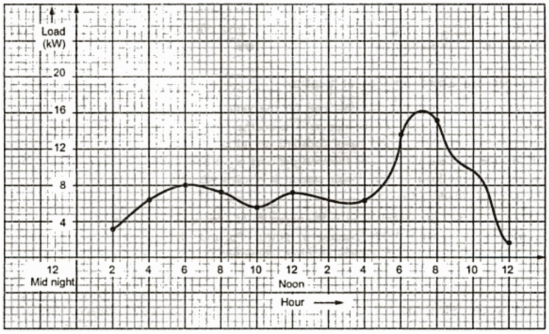We have already seen that the load on the power station goes on changing with respect to time. So if these variations are plotted then curve obtained is called load curve. From the load curve following information is obtained,
1. The variation of load on the plant during different hours of a day.
3. The maximum and minimum values of load during a day.
3. Maximum and minimum values of load during a year.
4. Average load on the station during a year.
5. It gives the indication whether the station is working efficiently or not.
The load curve may be a daily, monthly or yearly on the basis of whether the load variation are plotted during a day, month or a year.
In a daily load curve, the load variations are plotted against time on graph after recording their values on half-hourly or hourly basis. Fig.1 Shows a typical load curve on the plant. From this figure the general character of the load can be obtained which is not possible with tabulated values.Fig. 1 Typically daily load curve

It can be seen from the above curve that the maximum load occurs during the period between 6 p.m. to 8 p.m. whereas it is minimum in the afternoon period.
The monthly load curve can be obtained from daily load curve of that month. The average values of load at different periods of the day for the month is calculated and then the curve is plotted on the graph. This curve helps in determining the rates of energy.
The yearly load curve can be obtained from monthly load curve of that year. The annual load factor can be determined from this curve.
Now consider the above daily load curve. The area under this curve gives the number of units generated in the day.
Units generated in a day = Area under daily load curve.
The highest point on the load curve indicates the maximum demand on the station for that day.
If the area under this curve is divided by total number of hours gives the average load on the station.

The load factor can also be calculated by taking a ratio of area under load curve to the total area of the rectangular in which the curve is contained.

The size and generating units cab be obtained from the load curve. The number of generating units are such as to fit the load curve. This aids in operating the generating units near or at maximum efficiency.
Depending on load demands obtained from the load curve, the generating units can be put in operation. This sequence and time for which units are operated can be decided from the information obtained from load curve. Thus the operating schedule of the plant can be operated from load curve.

Before plotting the load curve, the load is divided into number of categories. The load is classified into following different categories.
4) Shops, hostels and hospital load
8) Street light.
The load sheet is prepared for each locality. Then the total of each type of load in different hours in a day are obtained.
The reading taken for each types of load during different hours of a day are plotted on a graph which gives the load curve.
The load curve goes on changing from summer to winter. In the summer season, there is a load of refrigerators, fan and air conditioning. As the light load comes up after sunset the maximum demand of load will be between 8.00 p.m. to 10.0 p.m. In winter the load conditions are different and correspondingly peak demand are different.
The typical load curves for Delhi in summer, winter and monsoon season are shown in following Fig.2, 3, and 4.Fig. 2 Winter seasonFig. 3   Summer seasonFig. 4   Monsoon season

For each category of load, the load curve may be plotted. The summation of all these points gives the total load curve.
The maximum demand on the generating station can be obtained from the load curve for the given period. The maximum demand is nothing but the maximum load on the station during a given period. The peak load obtained from thew load curve is not the maximum demand. The maximum demand is the largest average load on the station for specified period. If the load readings are monitored continuously then the greatest load will be represent instantaneous maximum demand.
It is stated that the maximum demand is x kW, then it is meaningless unless it is specified by the time duration. The accuracy of this will dependent on how accurately the readings are obtained from load curves, scale of the curve and the number of ordinates taken within the specified period. The capacity of generating units can be decided from this information.